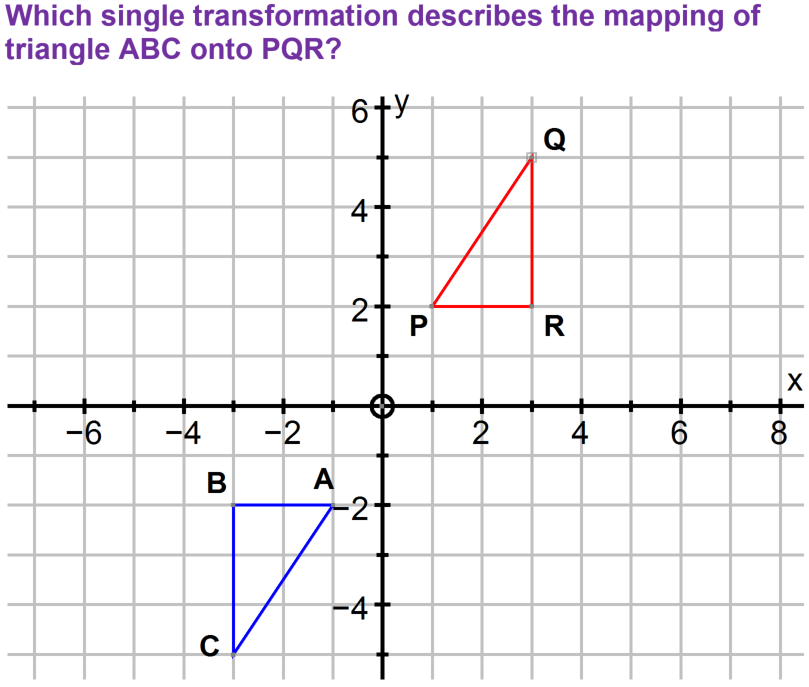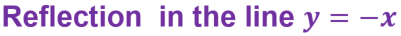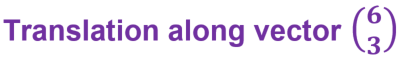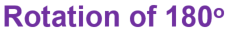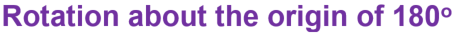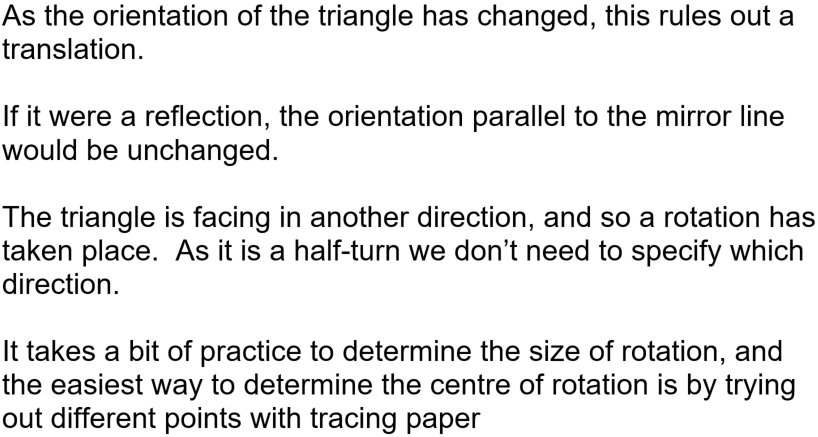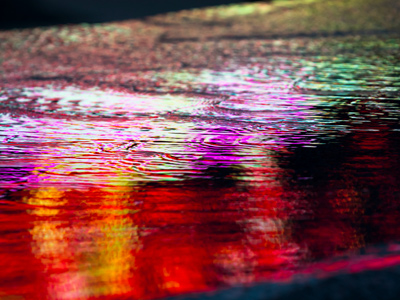This is one type of reflection but you will be dealing with another type in this quiz.

# Reflections, Translations and Rotations (F)

There are a number of ways in which we can TRANSFORM, or change, an object. Three of these, TRANSLATION, REFLECTION and ROTATION will result in the image (the transformed shape) being congruent to the object (the original shape). The fourth transformation, ENLARGEMENT, does what it says, and will produce an image similar to the object. If you’re not sure what congruent and similar means in a mathematical context, try the Mathematical Definitions quizzes first, before testing yourself in this GCSE Maths quiz.

Translating an object means to slide it, without twisting it (rotating) or turning it over (reflecting). To describe a translation you need to know how far left or right to move it, and how far up or down. This information is often presented as a VECTOR.

When we rotate an object, we need to know three things: the centre of rotation, how far to turn (normally given as an angle), and a direction.

Just as you need a mirror to see your reflection, an object needs a mirror line to be able to reflect it. The mirror line could be given as an equation, so make sure you know how to draw a straight line! Common mirror lines are the axes, and the line y = x. Practise reflecting shapes in these lines and spot what happens to the coordinates.

Question 1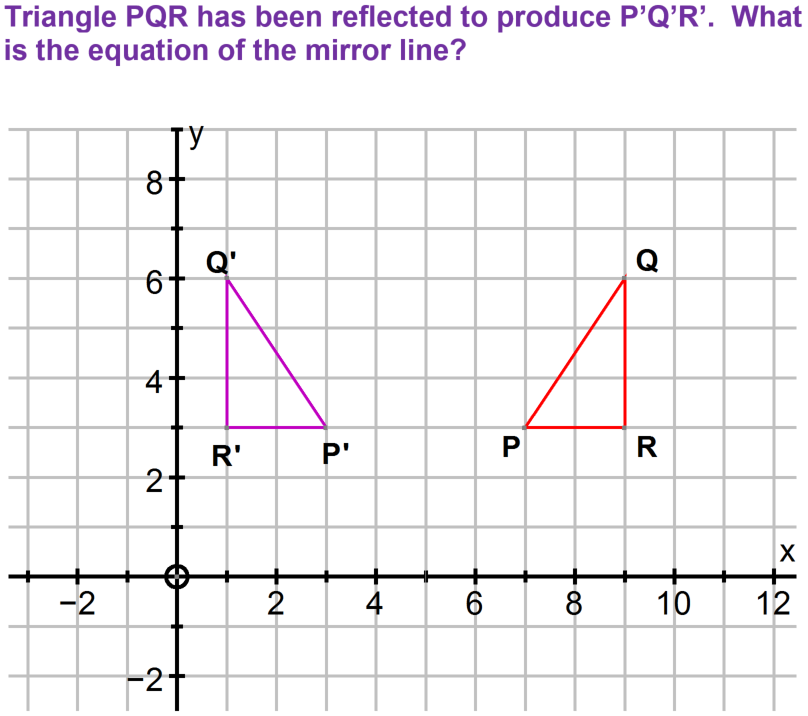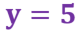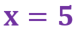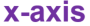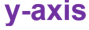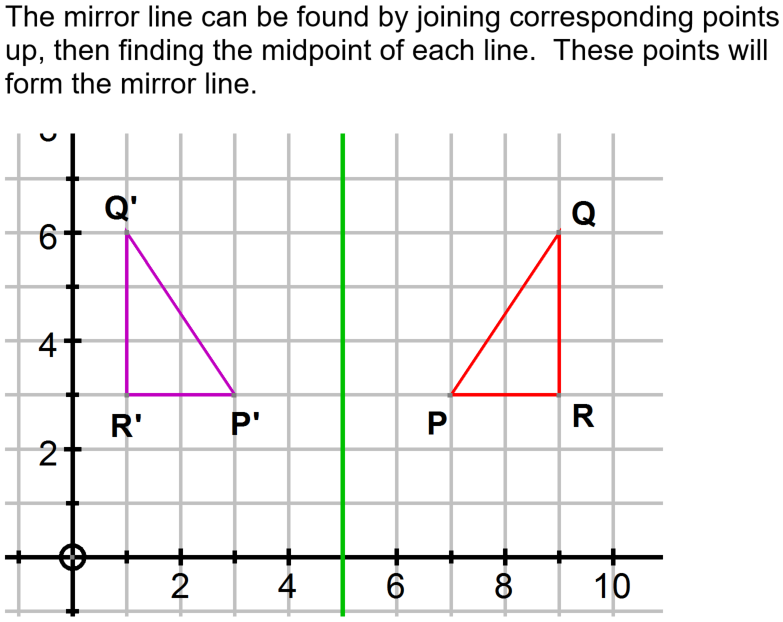Question 2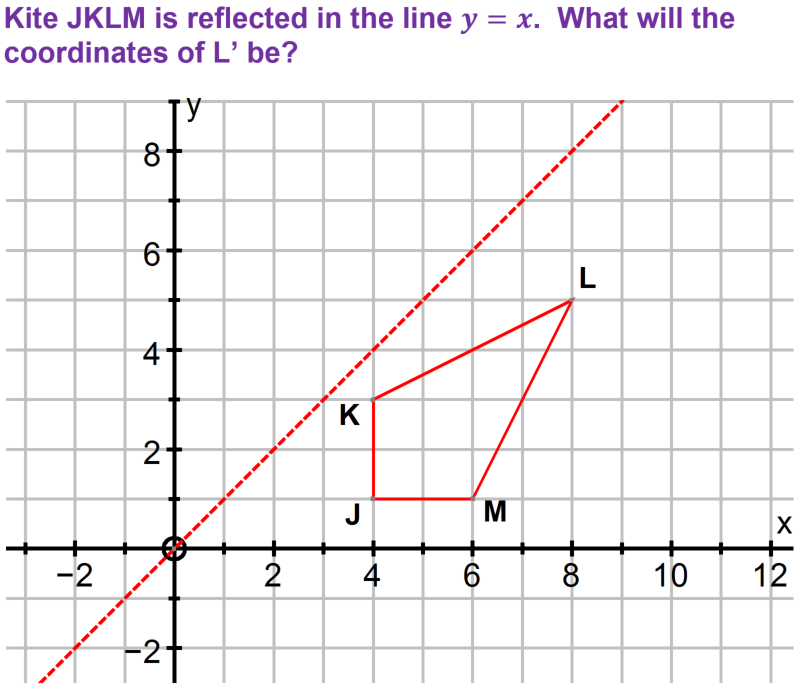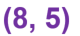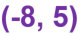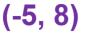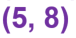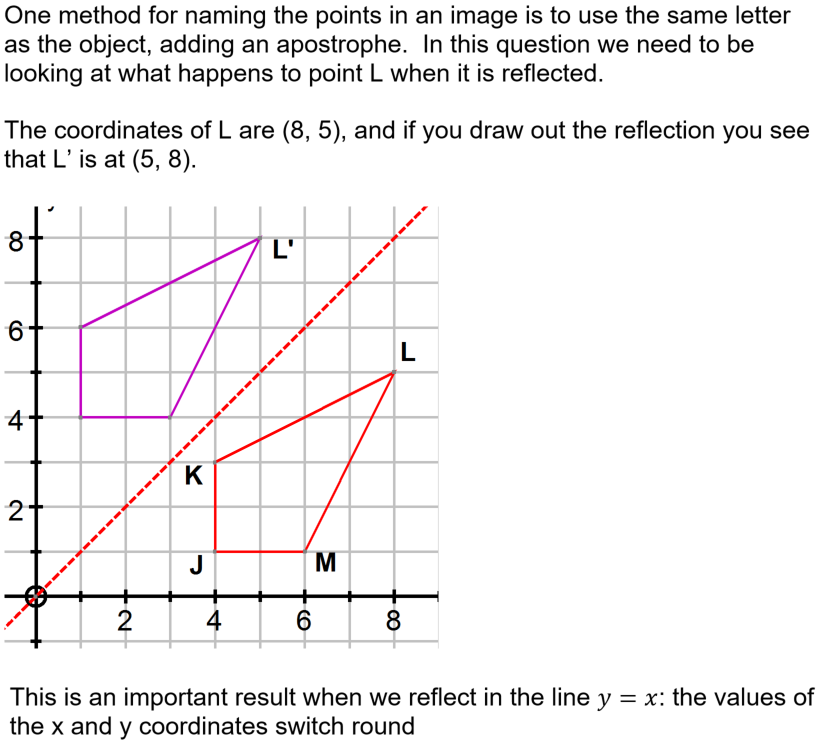Question 3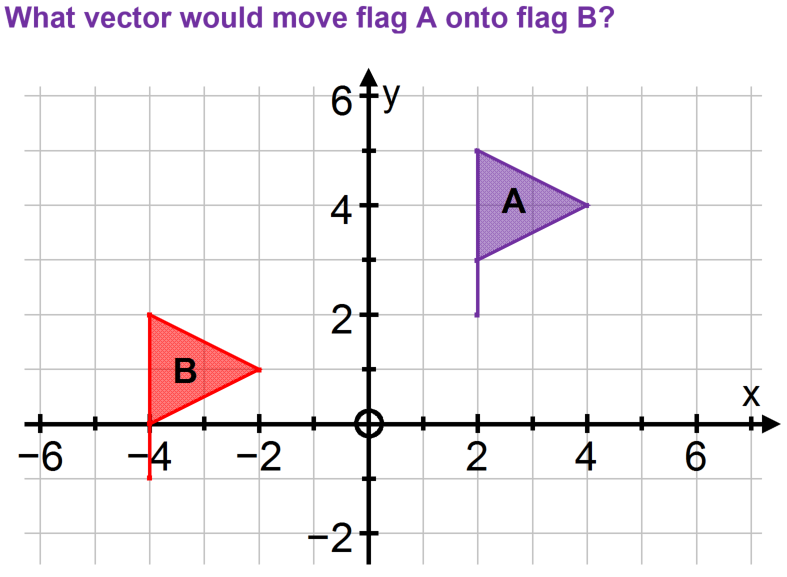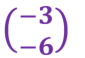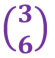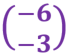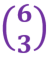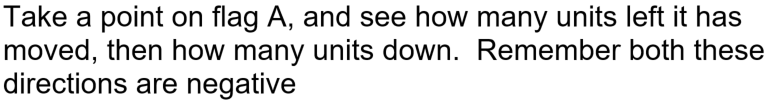Question 4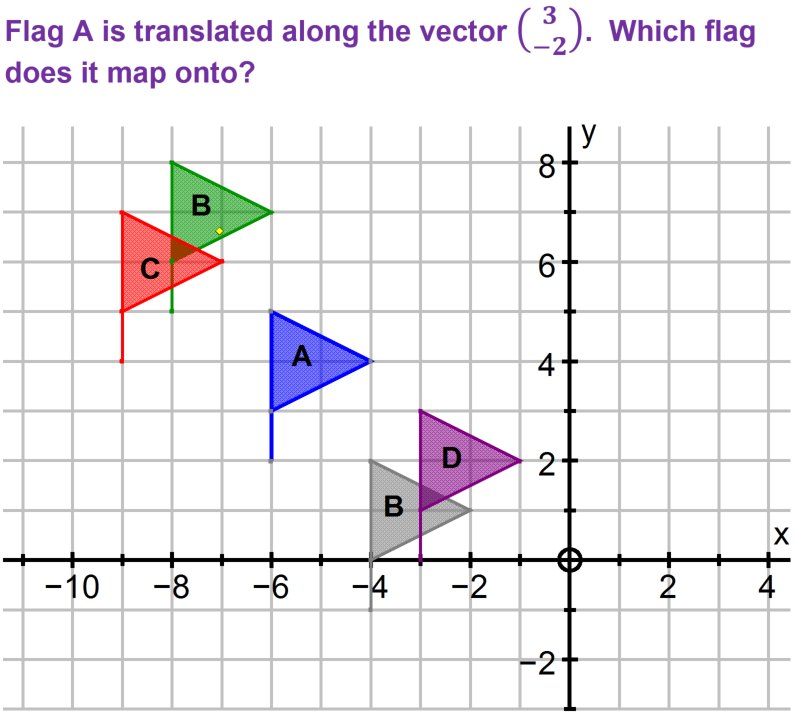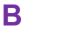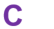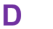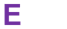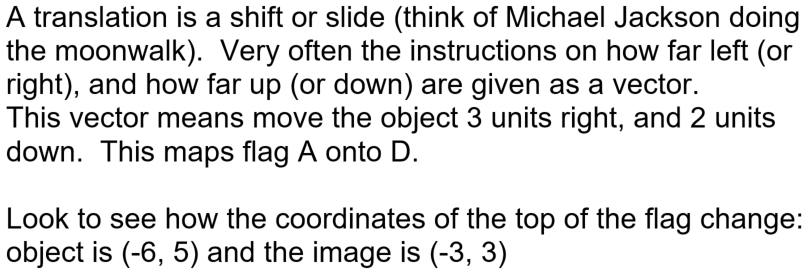Question 5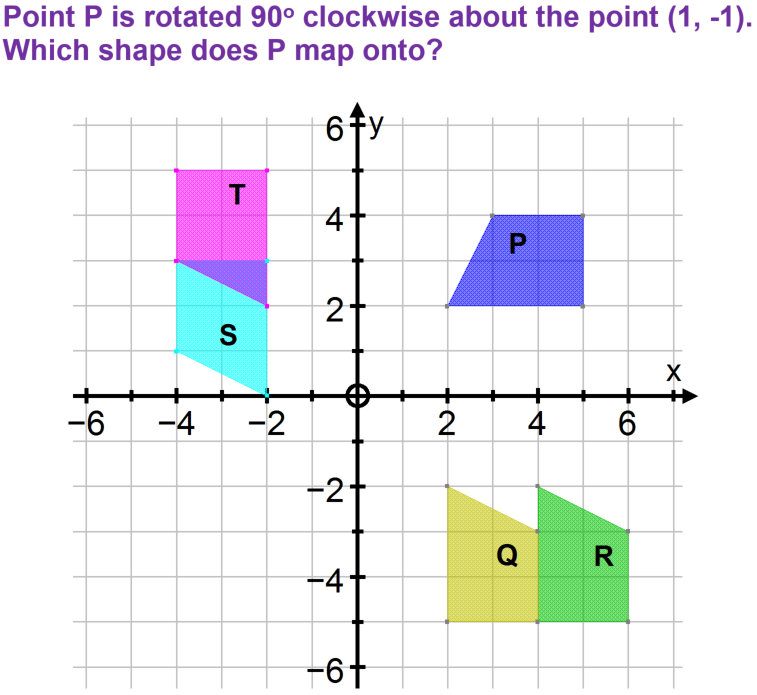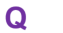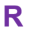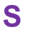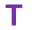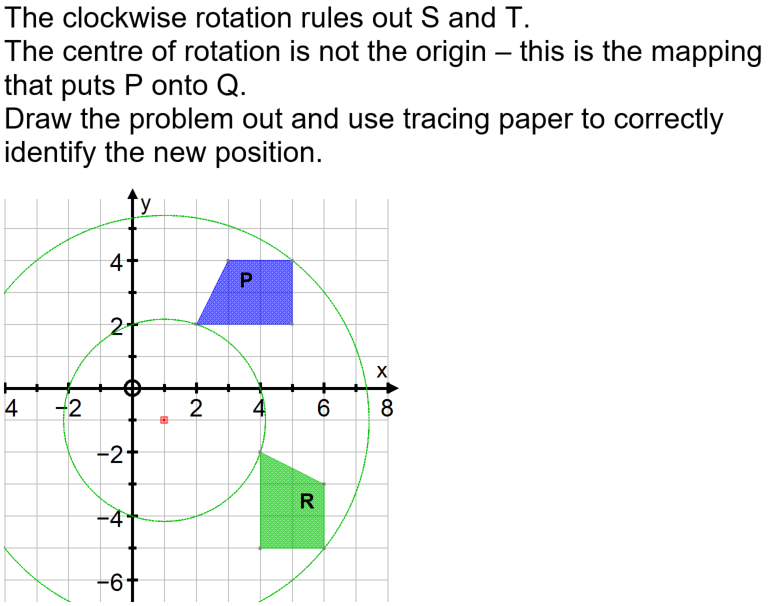Question 6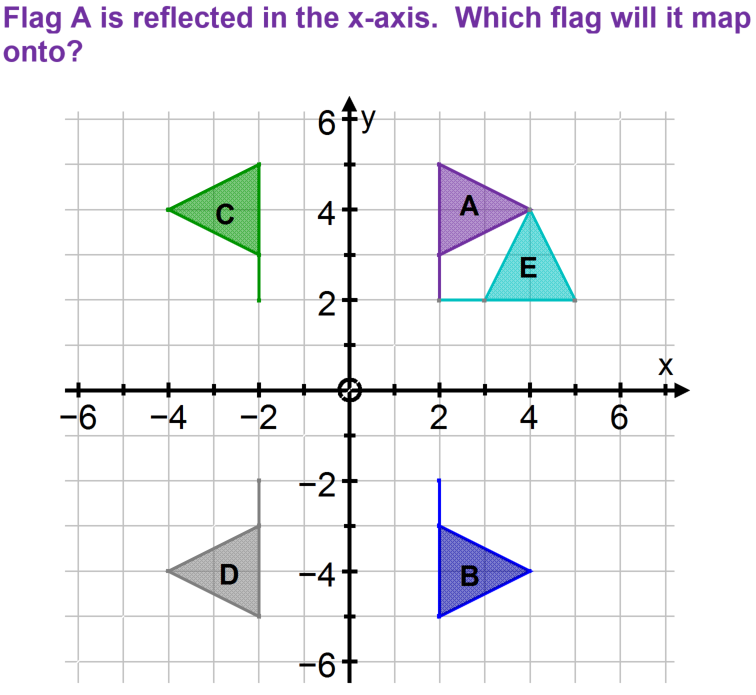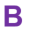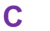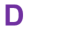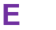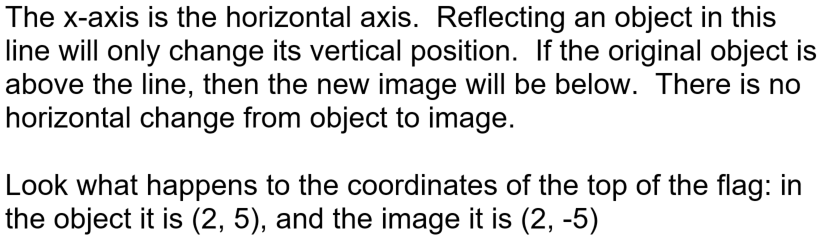Question 7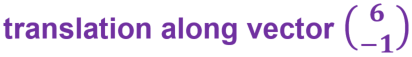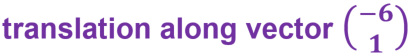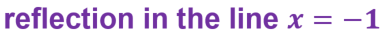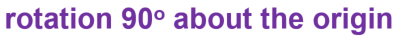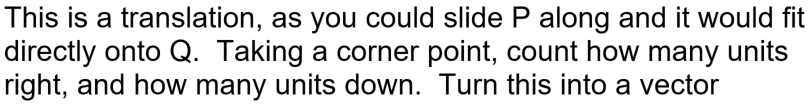Question 8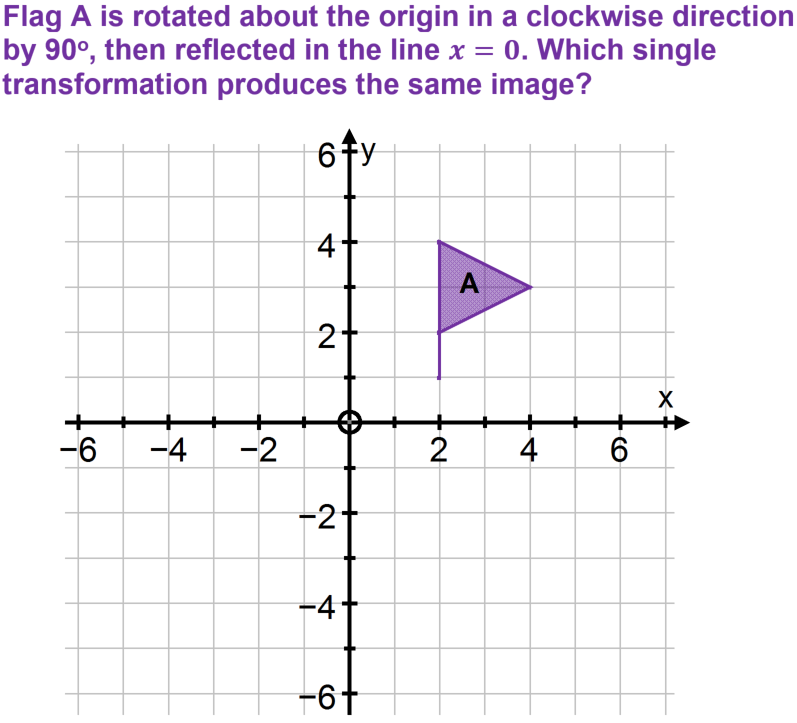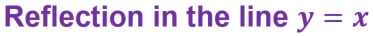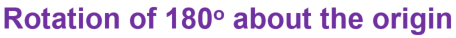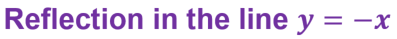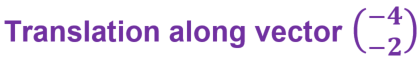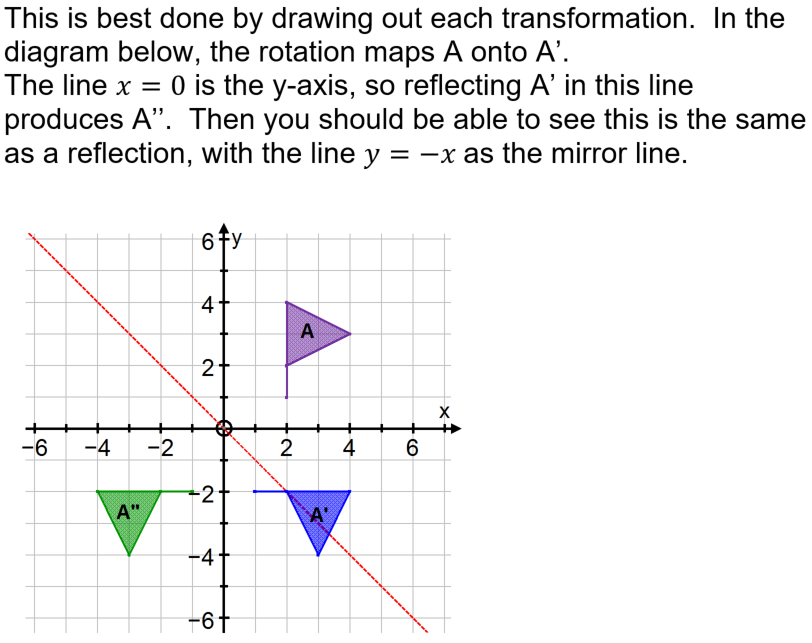Question 9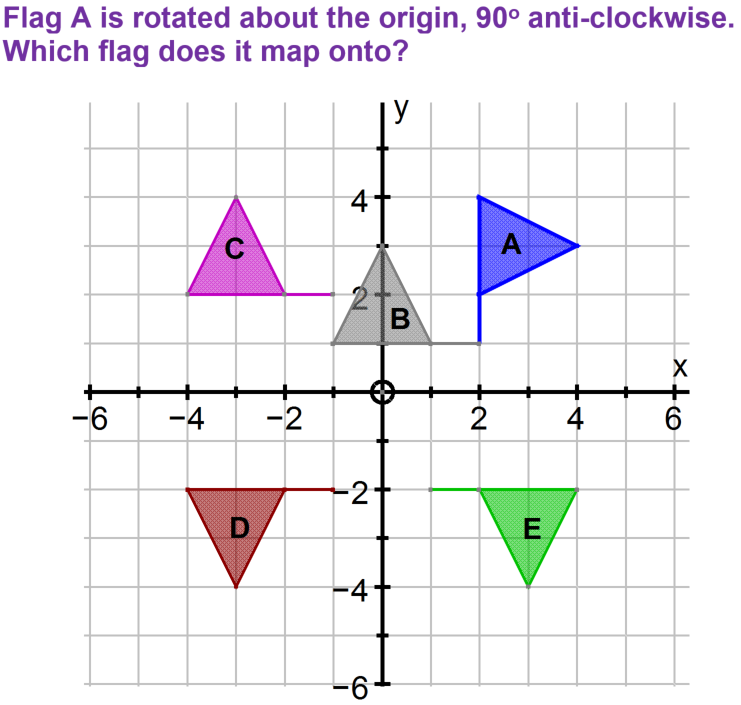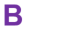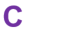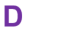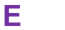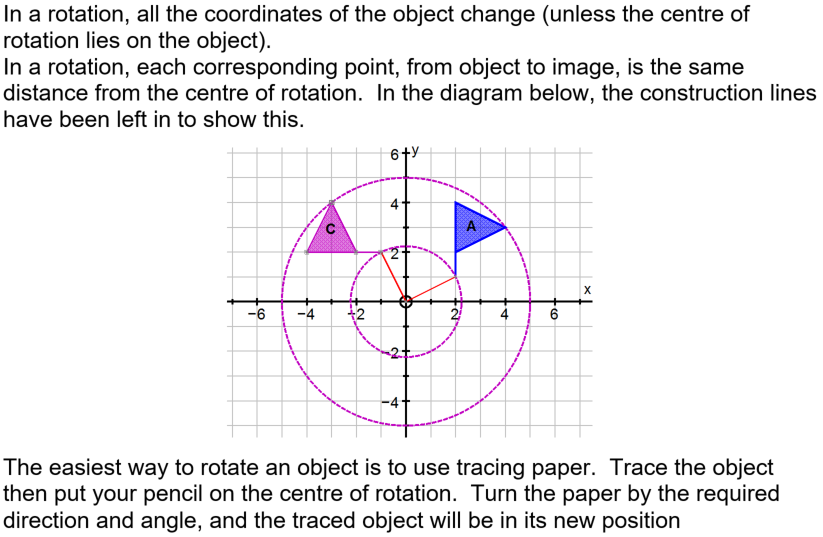Question 10